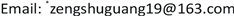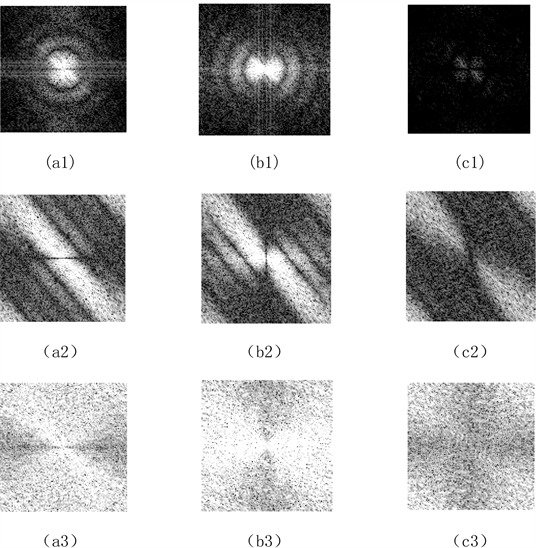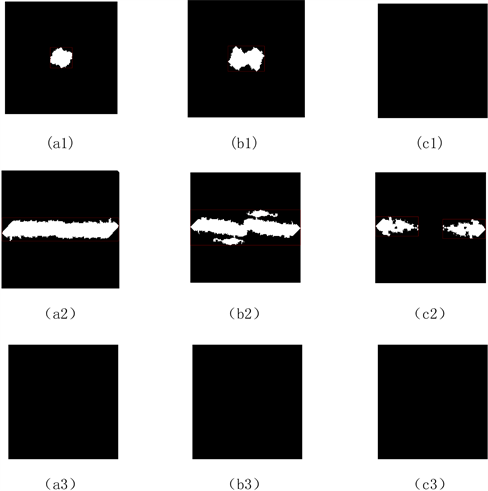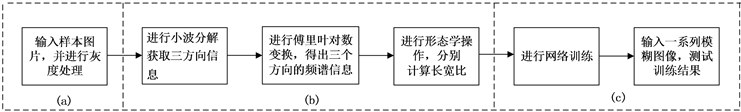﻿ 基于支持向量机的小波分解的图像模糊分类方法 An Image Fuzzy Classification Method Based on Support Vector Machine (SVM) Wavelet Decomposition

Journal of Image and Signal Processing
Vol. 09  No. 01 ( 2020 ), Article ID: 34003 , 7 pages
10.12677/JISP.2020.91009

An Image Fuzzy Classification Method Based on Support Vector Machine (SVM) Wavelet Decomposition

Mingxuan Cai, Xiaoxiong Zuo, Lei Liu, Lingli Huang, Shuguang Zeng*

College of Science, China Three Gorges University, Yichang HubeiReceived: Dec. 25th, 2019; accepted: Jan. 9th, 2020; published: Jan. 16th, 2020ABSTRACT

To solve the problem of fuzzy image classification, an image fuzzy classification method based on support vector machine (SVM) wavelet decomposition was proposed. After collecting the samples, firstly, the image is processed in grayscale, and then the image is decomposed in wavelet transform to obtain the horizontal, vertical and diagonal messages of the second layer. After that, Fourier logarithm transform is performed on the image to obtain the spectral information in three directions, and then appropriate threshold is used to carry out the spectral image. Then, the connected domain border markers in the spectrum are processed, the aspect ratio is calculated, and the aspect ratio is taken as the training standard for network training. The experimental results show that this method can greatly reduce the computation of fuzzy image classification and has high accuracy in processing and classifying fuzzy images.

Keywords:Fuzzy Image Classification, Support Vector Machines, Morphological Operations, Wavelet Decomposition1. 引言

2. 数字图像的小波变换与模糊图像的频域特征

2.1. 图像特征

${L}^{2}\left(R\right)$ 空间的任意函数 $f\left(t\right)$ 在小波基下展开，小波变换式为  ：

$W{T}_{f}\left(a,\tau \right)=\frac{1}{\sqrt{a}}\int f\left(t\right)\psi \left(\frac{t-\tau }{a}\right)\text{d}t$

1. 把小波w(t)和原函数f(t)的开始部分进行比较，计算系数C。系数C表示该部分函数与小波的相似程度。

2. 把小波向右移k单位，得到小波w(t − k)，重复1。重复该步骤直至函数f结束。

3. 扩展小波w(t)，得到小波w(t/2)，重复步骤1，2。

4. 不断扩展小波，重复1，2，3。Figure 1. (a1) Defocus fuzzy image level information; (b1) Vertical information of defocused fuzzy image; (c1) Diagonal information of defocused fuzzy image; (a2) Horizontal information of moving fuzzy image; (b2) Vertical information of moving fuzzy image; (c2) Diagonal information of moving fuzzy image; (a3) Normal image level information; (b3) Vertical information of normal image; (c3) Diagonal information of normal image

2.2. 模糊分类方法Figure 2. (a1) Block diagram of horizontal connected domain of defocused fuzzy image; (b1) Block diagram of vertically connected domain of defocused fuzzy image; (c1) Block diagram of diagonally connected domain of defocused fuzzy image; (a2) Block diagram of vertically connected domain of moving fuzzy image; (b2) Block diagram of horizontally connected domain of moving fuzzy image; (c2) Diagonally connected domain diagram of moving fuzzy image; (a3) Block diagram of horizontally connected domain of normal image; (b3) Block diagram of vertically connected domain of normal image; (c3) Diagonally connected domain block diagram of normal image

2.3. 支持向量机基本原理

$f\left(x\right)=\omega \varphi \left(x\right)+b$

$\mathrm{min}\text{ }\text{ }\text{ }\text{ }\text{ }\frac{1}{2}{‖\omega ‖}^{2}+C\underset{i=1}{\overset{l}{\sum }}\left({\xi }_{i}+{\xi }_{i}{}^{*}\right)$

$s.t.\left\{\begin{array}{l}{y}_{i}-\left(\omega \varphi \left(x\right)+b\right)\le \epsilon +{\xi }_{i}\\ -{y}_{i}+\left(\omega \varphi \left(x\right)+b\right)\le \epsilon +{\xi }_{i}{}^{*}\\ {\xi }_{i},{\xi }_{i}{}^{*}\ge 0,i=1,2,\cdots l\end{array}$ (3)

$\mathrm{min}\text{ }\text{ }\text{ }D=\text{ }\text{ }\text{ }\frac{1}{2}\underset{i=1}{\overset{l}{\sum }}\underset{j=1}{\overset{l}{\sum }}\left({\alpha }_{i}-{\alpha }_{i}^{*}\right)\left({\alpha }_{j}-{\alpha }_{j}^{*}\right)K\left({x}_{i},{x}_{j}\right)+\underset{i=1}{\overset{l}{\sum }}\left({\alpha }_{i}+{\alpha }_{i}^{*}\right)\epsilon -\underset{i=1}{\overset{l}{\sum }}\left({\alpha }_{i}-{\alpha }_{i}^{*}\right){y}_{i}$

$s.t.\left\{\begin{array}{l}\underset{i=1}{\overset{l}{\sum }}\left({\alpha }_{i}-{\alpha }_{i}^{*}\right)=0\\ 0\le {\alpha }_{i},{\alpha }_{i}^{*}\le C\end{array}$

3. 算法流程Figure 3. Algorithm flow chart

1) 采集样本图片，修改尺寸为500 × 500并输入其灰度值图像。如图3(a)所示。

2) 进行小波变换分解得到水平、垂直、对角三个方向上的图像信息，然后再分别对分解后的图像进行傅里叶变换，经过对数变换和中心处理后得到合适的傅里叶频谱图，再进行二值化处理，得到三种模糊更加明显的特征信息。

3) 对处理后的频谱图进行形态学操作，以消除部分无关信息的干扰。最后得到连通域较大的频谱特征区域。

4) 计算该连通区域的长宽比，定义 $\partial ={l}_{c}/{l}_{k}$，如果有多个连通区域则进行近似拼接,计算拼接后连通区域的长宽比。若没有连通区域定义为0。如图3(b)所示。

5) 建立以支持向量机的网络训练模型。将同一张图像在三个不同方向上的长宽比 ${\partial }_{1},{\partial }_{2},{\partial }_{3}$ 作为网络输入层，再将离焦模糊定义为1，运动模糊图像定义为2，正常图片定义为3，将这三种分类作为网络输出层，最终可以形成一个100 × 4的训练矩阵，其中前3列为网络输入，最后1列为网络输出。最后将训练样本进行网络训练。

6) 输入一系列模糊图像，得到最终的结果。如图3(c)所示。

4. 实验结果Figure 4. Two random blur images and normal image

5. 结论

An Image Fuzzy Classification Method Based on Support Vector Machine (SVM) Wavelet Decomposition[J]. 图像与信号处理, 2020, 09(01): 71-77. https://doi.org/10.12677/JISP.2020.91009

1. 1. 毛建旭, 王耀南, 孙炜. 一种基于模糊小波基函数神经网络的图像分类器[J]. 仪器仪报, 2003(2): 114-118.

2. 2. 林光忠, 张超兰, 周俊, 曾曙光. 奇异值分解与傅里叶变换相结合的模糊图像分类方法[J]. 图像与信号处理, 2019, 8(2): 36-42.

3. 3. 禹晶. 散焦与运动模糊图像参数辨识的研究[C]//中国图象图形学学会. 第十三届全国图象图形学学术会议论文集. 南京: 中国图象图形学学会, 2006: 5.

4. 4. 王冠军, 吴志勇, 云海姣, 梁敏华, 杨华. 结合图像二次模糊范围和奇异值分解的无参考模糊图像质量评价[J]. 计算机辅助设计与图形学学报, 2016, 28(4): 653-661.

5. 5. 李涛. 基于卷积神经网络的图像分类模型的研究与应用[D]: [硕士学位论文]. 武汉: 湖北工业大学, 2018.

6. 6. Wu, Z.J. (2018) Image Classification Based on Support Vector Machines. Proceedings of 2018 8th International Conference on Education and Sports Education, Saint Julian’s, 22-24 August 2018, 8.

7. 7. Tiwari, S., et al. (2014) Blur Classification Using Ridgelet Transform and Feed Forward Neural Network. International Journal of Image, Graphics and Signal Processing, 9, 47-53.
https://doi.org/10.5815/ijigsp.2014.09.06

8. 8. 米晨, 汤秀芬, 魏凤兰. 基于Haar小波变换的图像分解与重构[J]. 实验室研究与探索, 2003(2): 78-81.

9. 9. 任鸿鹏. 基于傅里叶变换的MATLAB图像处理[J]. 科技资讯, 2019, 17(16): 11-12+14.

10. 10. 田万春, 张贵宇, 庹先国, 吴昊翰, 石小仕. 基于支持向量机的白酒上甑探汽方法研究[J/OL]. 食品与机械: 1-9. http://kns.cnki.net/kcms/detail/43.1183.TS.20191211.1426.008.html, 2019-12-18.

11. 11. Su, B., Lu, S. and Tan, C.L. (2011) Blurred Image Region Detection and Classification. Proceedings of the 19th ACM International Conference on Multimedia, New York, 28 November-1 December 2011, 1397-1400.
https://doi.org/10.1145/2072298.2072024

12. NOTES

*通讯作者。